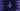# C itoa() function implementation explanation with example## C itoa() function implementation explanation with example:

In this post, we will learn what is itoa() function and how it works in C programming language. This function is used to convert int to string data type in C. This program will show you how to implement this function in C.

Let’s check the definition of itoa and its uses.

### Definition of itoa():

The itoa function is defined as like below:

``char* itoa(int n, char* buffer, int r)``

Here,

• n is the input integer. It converts that integer to a character string.
• buffer is the character pointer to hold the converted string.
• r is used for radix. It can be octal, decimal or hexadecimal.

• For decimal radix, the result is similar to `(void) sprintf(buffer, "%d", n)`
• For octal radix, it formats the value to an unsigned octal.
• For hexadecimal radix, it formats it to an unsigned hexadecimal constant.

### Return value of itoa():

It converts the integer to string and returns the string pointer.

### Why you need to implement itoa function:

The itoa function is not available by default in most common compilers in C. Instead, we can implement this function directly. Let me show you how:

``````#include <stdio.h>

void strrev(char *arr, int start, int end)
{
char temp;

if (start >= end)
return;

temp = *(arr + start);
*(arr + start) = *(arr + end);
*(arr + end) = temp;

start++;
end--;
strrev(arr, start, end);
}

char *itoa(int number, char *arr, int base)
{
int i = 0, r, negative = 0;

if (number == 0)
{
arr[i] = '0';
arr[i + 1] = '\0';
return arr;
}

if (number < 0 && base == 10)
{
number *= -1;
negative = 1;
}

while (number != 0)
{
r = number % base;
arr[i] = (r > 9) ? (r - 10) + 'a' : r + '0';
i++;
number /= base;
}

if (negative)
{
arr[i] = '-';
i++;
}

strrev(arr, 0, i - 1);

arr[i] = '\0';

return arr;
}

int main()
{
char buffer[sizeof(int) * 10 + 1];

printf("Enter a number: ");
scanf("%d", &no);

printf("Enter the radix value: ");

printf("itoa result %s\n", buffer);

return 0;
}``````
• In this program, we have implemented itoa. The itoa function takes the number, a string and the radix value as parameters. It puts the result in the string.
• This program reads the number and stores it in the variable no. It also reads the radix value from the user.
• It calls itoa that fills the character array buffer.
• The last line is printing the value of buffer.

If you run this program, it will print output as like below:

``````Enter a number: -120
Enter the radix value: 10
itoa result -120

Enter a number: 120
Enter the radix value: 2
itoa result 1111000

Enter a number: 3345
Enter the radix value: 16
itoa result d11``````